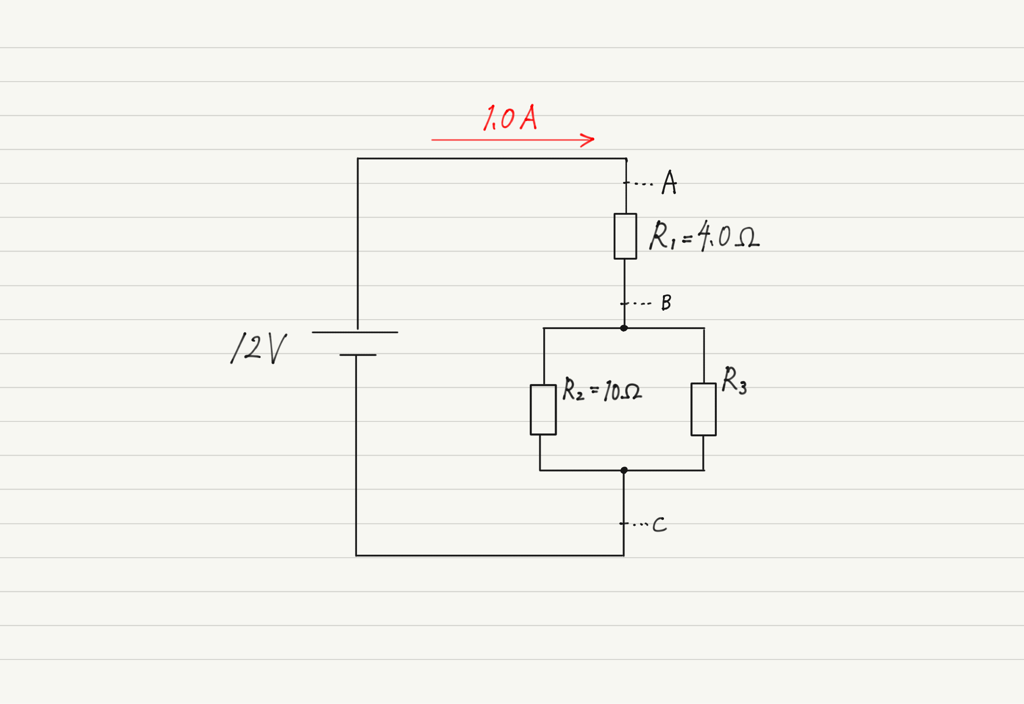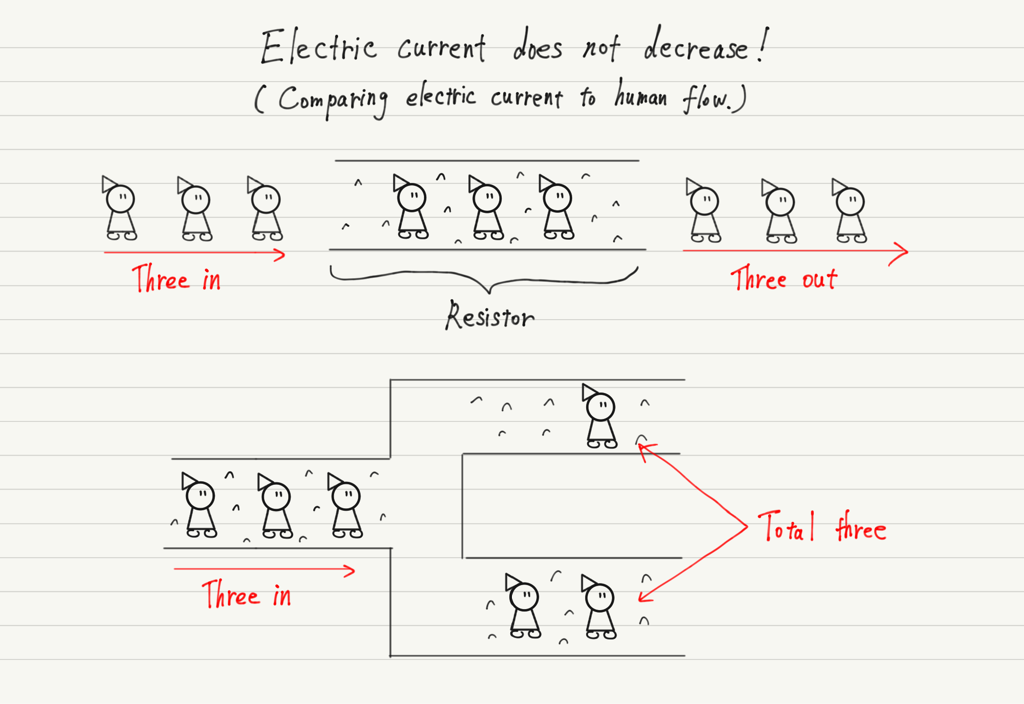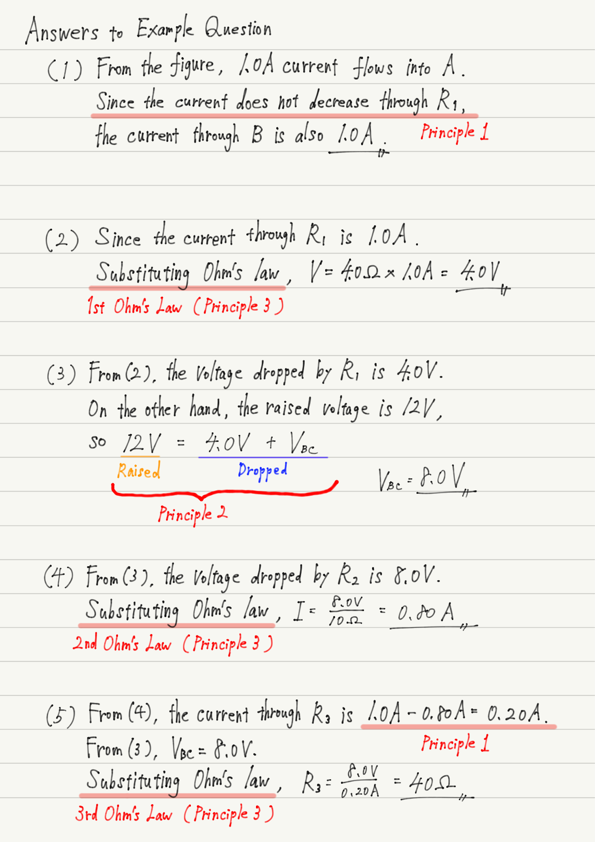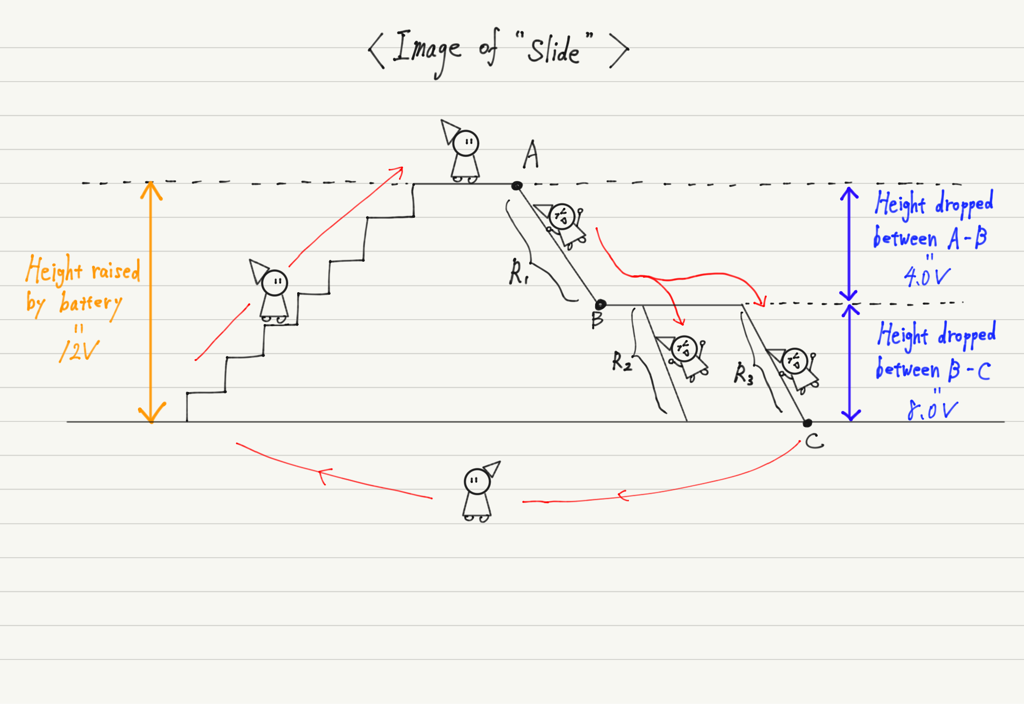# How to Apply Ohm’s Law

When solving an electric circuit problem, the first thing that comes to mind is Ohm’s law.
However, knowing Ohm’s law is not the only way to solve a problem. In this lecture, I will teach how to apply Ohm’s law to electric circuits using examples.

## Try the example

【Question】 Answer the following questions for the electric circuit shown below.

(１) Find the magnitude of the electric current flowing through B.

(２) Find the voltage between A-B.

(３) Find the voltage between B-C.

(４) Find the magnitude of the electric current flowing through resistor 2.

(５) Find the resistance of R3.The following are some tips for solving electric circuit problems. If you cannot solve this problem on your own, please refer to the tips.

## Principles in Electrical Circuits

There are three principles in solving electric circuit problems. They relate to electric current, voltage, and resistance.

1. The amount of current flowing through the circuit does not increase or decrease.

Some people think that “the current decreases as it passes through a resistance”, but they are wrong. The image of resistance is “an impassable road”, not “an impassable road”!
The current does not change before or after passing through the resistor because all the current will eventually pass through, even if it is on a path that is difficult to pass through.

The total current at the end of the branch is the same as before the branching, even though the circuit may be branched in the middle, as in a parallel circuit.2. The voltage raised by the battery is equal to the voltage lowered by the resistor.

This is explained in the lecture on voltage, so it is omitted here.

https://www.yukimura-physics.com/entry/elemag-f06

3. Use Ohm’s law for the number of resistors in the circuit.

Ohm’s law is not a law for the entire circuit, but for each resistance.

So if there are multiple resistors in a circuit, Ohm’s law can be used for each one. Since there are three resistors in this problem, let’s approach the problem thinking that Ohm’s law is used three times.

Below are the answers to the example questions.Did you solve problem (3)? If you were unable to do so, try to imagine the circuit in question as a “slide”.Memorization of formulas will not help you apply them, so try to visualize the circuit!
The important aspect of the example circuit is that the slide is going down twice.

Then, in (4), where Ohm’s law is used, some of you may have substituted the battery voltage of 12 V for V in your calculations.

Substituting for V in Ohm’s law is not the voltage of the battery, but the voltage dropped by the resistor!

Once you have an image of the circuit in your mind, you can solve any problem using the three principles. Please try many problems and develop your problem-solving skills.

## Next Time

In the next lecture, we will look at the phenomenon of heat generation when a current flows through a resistor.

https://www.yukimura-physics.com/entry/elemag-f09

error: Content is protected !!